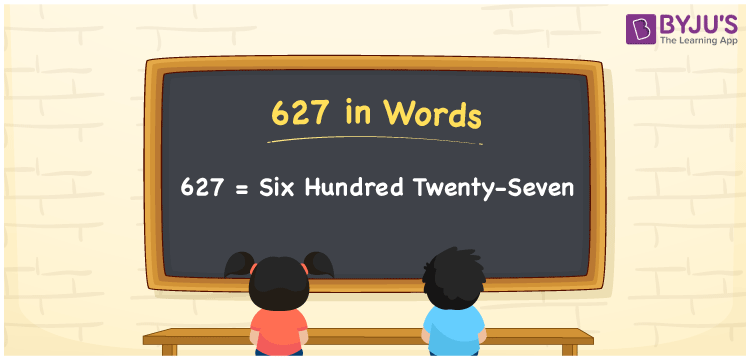# 627 in Words

627 in words is written as Six Hundred Twenty-seven. The name of number 627 in English is “Six Hundred Twenty-seven”. The word Six Hundred Twenty-seven is used in many real-life situations. For example, there are Six Hundred Twenty-seven people in a market. Thus, it is a counting number. Also, to represent the currency equal to 627, we can write it in words as Rupees Six Hundred Twenty-seven or Six Hundred Twenty-seven rupees. Therefore, it is necessary to learn the numbers in words, for the ease of understanding and expressing them.

 627 in words Six Hundred Twenty-seven Six Hundred Twenty-seven in Numbers 627

## 627 in English Words## How to Write 627 in Words?

If we know the place value of digits of 627, then we can easily express it in words. The place value is basically the position of a digit in a number. 627 is a three-digit number, therefore, we can specify the position of each digit of 627 in a place value chart. In the Indian numbering system, the order of place value of digits from right to left is given by:

 Hundreds Tens Ones 6 2 7

We can write it in expanded form as:

6 × Hundred + 2 × Ten + 7 × One

= 6 × 100 + 2 × 10 + 7 × 1

= 600 + 20 + 7

= 627

= Six Hundred Twenty-seven

Therefore, 627 in words is written as Six Hundred Twenty-seven.

627 is a natural number that precedes 628 and succeeds 626.

627 in words – Six Hundred Twenty-seven

Is 627 an odd number? – Yes

Is 627 an even number? – No

Is 627 a perfect square number? – No

Is 627 a perfect cube number? – No

Is 627 a prime number? – No

Is 627 a composite number? – Yes

## Frequently Asked Questions on 627 in Words

Q1

### How to write 627 in words?

627 in English is written as “Six Hundred Twenty-seven”.
Q2

### Is 627 an odd number or even number?

627 is an odd number because it is not wholly divisible by 2.
Q3

### Write Six Hundred Twenty-seven in numbers.

Six Hundred Twenty-seven in numbers is 627.